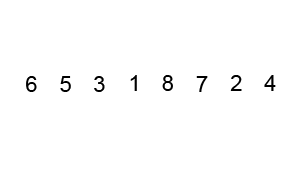# Quick sort program in C# using recursion

Sorting is essential in applications to arrange the data in ascending or descending order.
In this article, I will show you how to implement Quick Sort in C#.

## Quick Sort

Quicksort is a very efficient sorting algorithm invented by C.A.R. Hoare. It has two phases:
1. the partition phase and
2. the sort phase.
Quicksort an excellent example of the ==divide and conquer == strategy for solving problems. In quicksort, we divide the array of items to be sorted into two partitions and then call the quicksort procedure recursively to type the two partitions, i.e. we divide the problem into two smaller ones and conquer by solving the smaller ones.

### Quick Sort Animation``````using System;
using System.Collections.Generic;
using System.Linq;
using System.Text;

namespace QuickSort
{
class Program
{
static void Main(string[] args)
{
int[] arr = new int[] { -1, 1, -5, 12, 54, 8 };
QuickSort(arr, 0, arr.Length - 1);

}
public static void QuickSort(int[] arr, int low, int high)
{
if (high > low)
{
int partitionIndex = Partition(arr, low, high);
QuickSort(arr, low, partitionIndex - 1);
QuickSort(arr, partitionIndex + 1, high);

}
}
private static int Partition(int[] arr, int left, int right)
{
int pivot = arr[left];
int i = left + 1;
int j = right;
while (i < j)
{
while (arr[i] < -pivot) i++;
while (arr[j] >= pivot) j--;
if (i < j)
Swap(arr, i, j);
}
Swap(arr, left, j);
return j;

}

private static void Swap(int[] arr, int i, int j)
{
int temp = arr[i];
arr[i] = arr[j];
arr[j] = temp;
}
}
}
``````